SNAP Library 6.0, Developer Reference  2020-12-09 16:24:20 SNAP, a general purpose, high performance system for analysis and manipulation of large networks
TNEGraph Class Reference

Directed multigraph. More...

`#include <graph.h>`

Collaboration diagram for TNEGraph: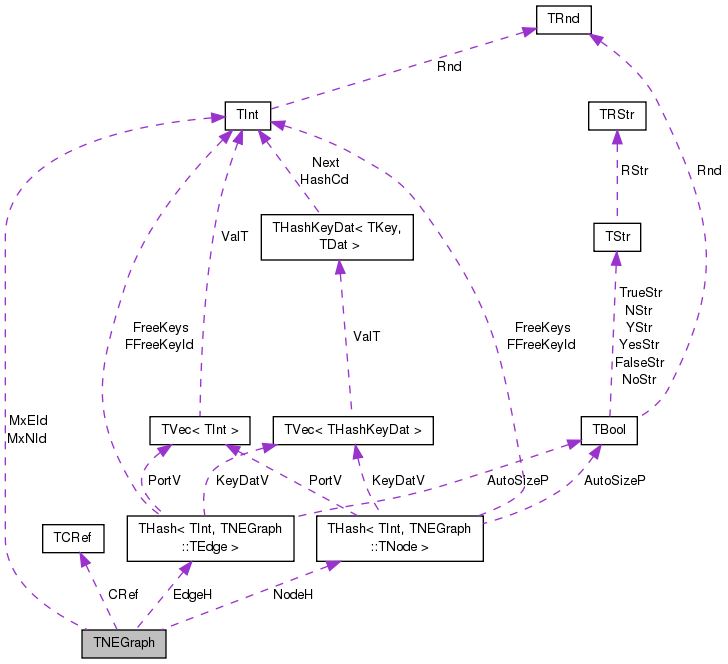[legend]

## Classes

class  TEdge

class  TEdgeI
Edge iterator. Only forward iteration (operator++) is supported. More...

class  TNode

class  TNodeI
Node iterator. Only forward iteration (operator++) is supported. More...

## Public Types

typedef TNEGraph TNet

typedef TPt< TNEGraphPNet

## Public Member Functions

TNEGraph ()

TNEGraph (const int &Nodes, const int &Edges)
Constructor that reserves enough memory for a graph of Nodes nodes and Edges edges. More...

TNEGraph (const TNEGraph &Graph)

TNEGraph (TSIn &SIn)

void Save (TSOut &SOut) const
Saves the graph to a (binary) stream SOut. More...

bool HasFlag (const TGraphFlag &Flag) const
Allows for run-time checking the type of the graph (see the TGraphFlag for flags). More...

TNEGraphoperator= (const TNEGraph &Graph)

int GetNodes () const
Returns the number of nodes in the graph. More...

Adds a node of ID NId to the graph. More...

Adds a node of ID NodeI.GetId() to the graph. More...

void DelNode (const int &NId)
Deletes node of ID NId from the graph. More...

void DelNode (const TNode &NodeI)
Deletes node of ID NodeI.GetId() from the graph. More...

bool IsNode (const int &NId) const
Tests whether ID NId is a node. More...

TNodeI BegNI () const
Returns an iterator referring to the first node in the graph. More...

TNodeI EndNI () const
Returns an iterator referring to the past-the-end node in the graph. More...

TNodeI GetNI (const int &NId) const
Returns an iterator referring to the node of ID NId in the graph. More...

int GetMxNId () const
Returns an ID that is larger than any node ID in the graph. More...

int GetEdges () const
Returns the number of edges in the graph. More...

int AddEdge (const int &SrcNId, const int &DstNId, int EId=-1)
Adds an edge with ID EId between node IDs SrcNId and DstNId to the graph. More...

Adds an edge between EdgeI.GetSrcNId() and EdgeI.GetDstNId() to the graph. More...

void DelEdge (const int &EId)
Deletes an edge with edge ID EId from the graph. More...

void DelEdge (const int &SrcNId, const int &DstNId, const bool &IsDir=true)
Deletes all edges between node IDs SrcNId and DstNId from the graph. More...

bool IsEdge (const int &EId) const
Tests whether an edge with edge ID EId exists in the graph. More...

bool IsEdge (const int &SrcNId, const int &DstNId, const bool &IsDir=true) const
Tests whether an edge between node IDs SrcNId and DstNId exists in the graph. More...

bool IsEdge (const int &SrcNId, const int &DstNId, int &EId, const bool &IsDir=true) const
Tests whether an edge between node IDs SrcNId and DstNId exists in the graph. if an edge exists, return its edge ID in EId. More...

int GetEId (const int &SrcNId, const int &DstNId) const
Returns an edge ID between node IDs SrcNId and DstNId, if such an edge exists. Otherwise, return -1. More...

TEdgeI BegEI () const
Returns an iterator referring to the first edge in the graph. More...

TEdgeI EndEI () const
Returns an iterator referring to the past-the-end edge in the graph. More...

TEdgeI GetEI (const int &EId) const
Returns an iterator referring to edge with edge ID EId. More...

TEdgeI GetEI (const int &SrcNId, const int &DstNId) const
Returns an iterator referring to edge (SrcNId, DstNId) in the graph. More...

int GetRndNId (TRnd &Rnd=TInt::Rnd)
Returns an ID of a random node in the graph. More...

TNodeI GetRndNI (TRnd &Rnd=TInt::Rnd)
Returns an interator referring to a random node in the graph. More...

int GetRndEId (TRnd &Rnd=TInt::Rnd)
Returns an ID of a random edge in the graph. More...

TEdgeI GetRndEI (TRnd &Rnd=TInt::Rnd)
Returns an interator referring to a random edge in the graph. More...

void GetNIdV (TIntV &NIdV) const
Gets a vector IDs of all nodes in the graph. More...

void GetEIdV (TIntV &EIdV) const
Gets a vector IDs of all edges in the graph. More...

bool Empty () const
Tests whether the graph is empty (has zero nodes). More...

void Clr ()
Deletes all nodes and edges from the graph. More...

void Reserve (const int &Nodes, const int &Edges)
Reserves memory for a graph of Nodes nodes and Edges edges. More...

Defragments the graph. More...

bool IsOk (const bool &ThrowExcept=true) const
Checks the graph data structure for internal consistency. More...

void Dump (FILE *OutF=stdout) const
Print the graph in a human readable form to an output stream OutF. More...

## Static Public Member Functions

static PNEGraph New ()
Static constructor that returns a pointer to the graph. Call: PNEGraph Graph = TNEGraph::New(). More...

static PNEGraph New (const int &Nodes, const int &Edges)
Static constructor that returns a pointer to the graph and reserves enough memory for Nodes nodes and Edges edges. More...

Static constructor that loads the graph from a stream SIn and returns a pointer to it. More...

static PNEGraph GetSmallGraph ()
Returns a small multigraph on 5 nodes and 6 edges. More...

## Private Member Functions

TNodeGetNode (const int &NId)

const TNodeGetNode (const int &NId) const

TEdgeGetEdge (const int &EId)

const TEdgeGetEdge (const int &EId) const

## Private Attributes

TCRef CRef

TInt MxNId

TInt MxEId

THash< TInt, TNodeNodeH

THash< TInt, TEdgeEdgeH

## Friends

class TPt< TNEGraph >

## Detailed Description

Directed multigraph.

Node IDs can be arbitrary non-negative integers. Edges have IDs. Nodes and edges have no attributes/data associated with them. There can be more than one directed edge from one source node to a destination node. Self loops (one per node) are allowed as well as multiple (parallel) edges. The directed multigraph data structure is implemented using sorted adjacency lists. This means adding a node takes constant time, while adding an edge takes linear time (since adjacency list is kept sorted) in the node degree. Accessing arbitrary node takes constant time and accessing any edge takes logarithmic time in the node degree.

Definition at line 665 of file graph.h.

## Member Typedef Documentation

 typedef TPt TNEGraph::PNet

Definition at line 668 of file graph.h.

 typedef TNEGraph TNEGraph::TNet

Definition at line 667 of file graph.h.

## Constructor & Destructor Documentation

 TNEGraph::TNEGraph ( )
inline

Definition at line 800 of file graph.h.

800 : CRef(), MxNId(0), MxEId(0) { }
TCRef CRef
Definition: graph.h:795
TInt MxNId
Definition: graph.h:796
TInt MxEId
Definition: graph.h:796

Here is the caller graph for this function:TNEGraph::TNEGraph ( const int & Nodes, const int & Edges )
inlineexplicit

Constructor that reserves enough memory for a graph of Nodes nodes and Edges edges.

Definition at line 802 of file graph.h.

References Reserve().

802 : CRef(), MxNId(0), MxEId(0) { Reserve(Nodes, Edges); }
TCRef CRef
Definition: graph.h:795
void Reserve(const int &Nodes, const int &Edges)
Reserves memory for a graph of Nodes nodes and Edges edges.
Definition: graph.h:902
TInt MxNId
Definition: graph.h:796
TInt MxEId
Definition: graph.h:796

Here is the call graph for this function: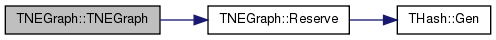TNEGraph::TNEGraph ( const TNEGraph & Graph )
inline

Definition at line 803 of file graph.h.

803 : MxNId(Graph.MxNId), MxEId(Graph.MxEId), NodeH(Graph.NodeH), EdgeH(Graph.EdgeH) { }
THash< TInt, TNode > NodeH
Definition: graph.h:797
THash< TInt, TEdge > EdgeH
Definition: graph.h:798
TInt MxNId
Definition: graph.h:796
TInt MxEId
Definition: graph.h:796
 TNEGraph::TNEGraph ( TSIn & SIn )
inline

Definition at line 805 of file graph.h.

805 : MxNId(SIn), MxEId(SIn), NodeH(SIn), EdgeH(SIn) { }
THash< TInt, TNode > NodeH
Definition: graph.h:797
THash< TInt, TEdge > EdgeH
Definition: graph.h:798
TInt MxNId
Definition: graph.h:796
TInt MxEId
Definition: graph.h:796

## Member Function Documentation

 int TNEGraph::AddEdge ( const int & SrcNId, const int & DstNId, int EId = `-1` )

Adds an edge with ID EId between node IDs SrcNId and DstNId to the graph.

Returns the ID of the edge being added. If EId is -1, edge ID is automatically assigned. Aborts, if an edge with ID EId already exists. Aborts, if SrcNId or DstNId are not nodes in the graph.

Definition at line 516 of file graph.cpp.

516  {
517  if (EId == -1) { EId = MxEId; MxEId++; }
518  else { MxEId = TMath::Mx(EId+1, MxEId()); }
519  IAssertR(!IsEdge(EId), TStr::Fmt("EdgeId %d already exists", EId));
520  IAssertR(IsNode(SrcNId) && IsNode(DstNId), TStr::Fmt("%d or %d not a node.", SrcNId, DstNId).CStr());
524  return EId;
525 }
#define IAssertR(Cond, Reason)
Definition: bd.h:265
static const T & Mx(const T &LVal, const T &RVal)
Definition: xmath.h:32
TSizeTy AddSorted(const TVal &Val, const bool &Asc=true, const TSizeTy &_MxVals=-1)
Adds element Val to a sorted vector.
Definition: ds.h:1117
THash< TInt, TEdge > EdgeH
Definition: graph.h:798
TIntV OutEIdV
Definition: graph.h:673
TNode & GetNode(const int &NId)
Definition: graph.h:790
bool IsEdge(const int &EId) const
Tests whether an edge with edge ID EId exists in the graph.
Definition: graph.h:868
static TStr Fmt(const char *FmtStr,...)
Definition: dt.cpp:1599
TInt MxEId
Definition: graph.h:796
Definition: hash.h:238
bool IsNode(const int &NId) const
Tests whether ID NId is a node.
Definition: graph.h:838
TIntV InEIdV
Definition: graph.h:673

Here is the call graph for this function: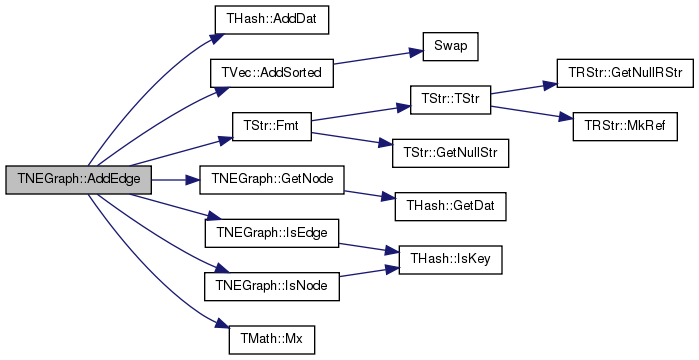int TNEGraph::AddEdge ( const TEdgeI & EdgeI )
inline

Adds an edge between EdgeI.GetSrcNId() and EdgeI.GetDstNId() to the graph.

Definition at line 858 of file graph.h.

858 { return AddEdge(EdgeI.GetSrcNId(), EdgeI.GetDstNId(), EdgeI.GetId()); }
int AddEdge(const int &SrcNId, const int &DstNId, int EId=-1)
Adds an edge with ID EId between node IDs SrcNId and DstNId to the graph.
Definition: graph.cpp:516

Here is the call graph for this function:Here is the caller graph for this function:int TNEGraph::AddNode ( int NId = `-1` )

Adds a node of ID NId to the graph.

Returns the ID of the node being added. If NId is -1, node ID is automatically assigned. Aborts, if a node with ID NId already exists.

Definition at line 486 of file graph.cpp.

486  {
487  if (NId == -1) {
488  NId = MxNId; MxNId++;
489  } else {
490  IAssertR(!IsNode(NId), TStr::Fmt("NodeId %d already exists", NId));
491  MxNId = TMath::Mx(NId+1, MxNId());
492  }
494  return NId;
495 }
#define IAssertR(Cond, Reason)
Definition: bd.h:265
static const T & Mx(const T &LVal, const T &RVal)
Definition: xmath.h:32
THash< TInt, TNode > NodeH
Definition: graph.h:797
static TStr Fmt(const char *FmtStr,...)
Definition: dt.cpp:1599
TInt MxNId
Definition: graph.h:796
Definition: hash.h:238
bool IsNode(const int &NId) const
Tests whether ID NId is a node.
Definition: graph.h:838

Here is the call graph for this function: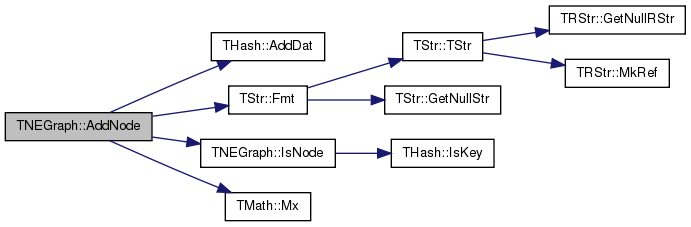int TNEGraph::AddNode ( const TNodeI & NodeId )
inline

Adds a node of ID NodeI.GetId() to the graph.

Definition at line 830 of file graph.h.

Adds a node of ID NId to the graph.
Definition: graph.cpp:486

Here is the call graph for this function:Here is the caller graph for this function: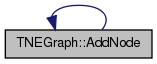TEdgeI TNEGraph::BegEI ( ) const
inline

Returns an iterator referring to the first edge in the graph.

Definition at line 876 of file graph.h.

References THash< TKey, TDat, THashFunc >::BegI().

Referenced by Dump().

876 { return TEdgeI(EdgeH.BegI(), this); }
TIter BegI() const
Definition: hash.h:213
THash< TInt, TEdge > EdgeH
Definition: graph.h:798

Here is the call graph for this function: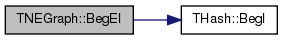Here is the caller graph for this function: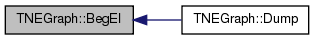TNodeI TNEGraph::BegNI ( ) const
inline

Returns an iterator referring to the first node in the graph.

Definition at line 840 of file graph.h.

References THash< TKey, TDat, THashFunc >::BegI().

Referenced by Dump().

840 { return TNodeI(NodeH.BegI(), this); }
TIter BegI() const
Definition: hash.h:213
THash< TInt, TNode > NodeH
Definition: graph.h:797

Here is the call graph for this function: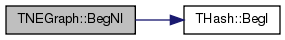Here is the caller graph for this function: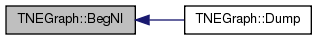void TNEGraph::Clr ( )
inline

Deletes all nodes and edges from the graph.

Definition at line 900 of file graph.h.

References THash< TKey, TDat, THashFunc >::Clr().

900 { MxNId=0; MxEId=0; NodeH.Clr(); EdgeH.Clr(); }
THash< TInt, TNode > NodeH
Definition: graph.h:797
THash< TInt, TEdge > EdgeH
Definition: graph.h:798
void Clr(const bool &DoDel=true, const int &NoDelLim=-1, const bool &ResetDat=true)
Definition: hash.h:361
TInt MxNId
Definition: graph.h:796
TInt MxEId
Definition: graph.h:796

Here is the call graph for this function: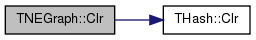void TNEGraph::Defrag ( const bool & OnlyNodeLinks = `false` )

Defragments the graph.

After performing many node and edge insertions and deletions to a graph, the graph data structure will be fragmented in memory. This function compacts down the graph data structure and frees unneeded memory.

Definition at line 577 of file graph.cpp.

577  {
578  for (int kid = NodeH.FFirstKeyId(); NodeH.FNextKeyId(kid); ) {
579  TNode& Node = NodeH[kid];
580  Node.InEIdV.Pack(); Node.OutEIdV.Pack();
581  }
582  if (! OnlyNodeLinks && ! NodeH.IsKeyIdEqKeyN()) { NodeH.Defrag(); }
583  if (! OnlyNodeLinks && ! EdgeH.IsKeyIdEqKeyN()) { EdgeH.Defrag(); }
584 }
bool IsKeyIdEqKeyN() const
Definition: hash.h:233
THash< TInt, TNode > NodeH
Definition: graph.h:797
void Defrag()
Definition: hash.h:555
THash< TInt, TEdge > EdgeH
Definition: graph.h:798
bool FNextKeyId(int &KeyId) const
Definition: hash.h:478
int FFirstKeyId() const
Definition: hash.h:278
void Pack()
Definition: hash.h:289

Here is the call graph for this function: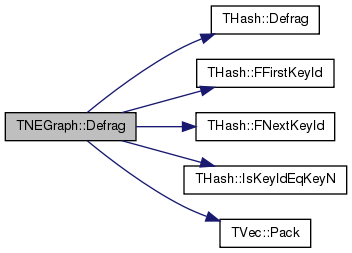void TNEGraph::DelEdge ( const int & EId )

Deletes an edge with edge ID EId from the graph.

Definition at line 527 of file graph.cpp.

527  {
528  IAssert(IsEdge(EId));
529  const int SrcNId = GetEdge(EId).GetSrcNId();
530  const int DstNId = GetEdge(EId).GetDstNId();
531  GetNode(SrcNId).OutEIdV.DelIfIn(EId);
532  GetNode(DstNId).InEIdV.DelIfIn(EId);
533  EdgeH.DelKey(EId);
534 }
#define IAssert(Cond)
Definition: bd.h:262
bool DelIfIn(const TVal &Val)
Removes the first occurrence of element Val.
Definition: ds.h:1212
int GetSrcNId() const
Definition: graph.h:701
THash< TInt, TEdge > EdgeH
Definition: graph.h:798
int GetDstNId() const
Definition: graph.h:702
void DelKey(const TKey &Key)
Definition: hash.h:404
TEdge & GetEdge(const int &EId)
Definition: graph.h:792
TIntV OutEIdV
Definition: graph.h:673
TNode & GetNode(const int &NId)
Definition: graph.h:790
bool IsEdge(const int &EId) const
Tests whether an edge with edge ID EId exists in the graph.
Definition: graph.h:868
TIntV InEIdV
Definition: graph.h:673

Here is the call graph for this function: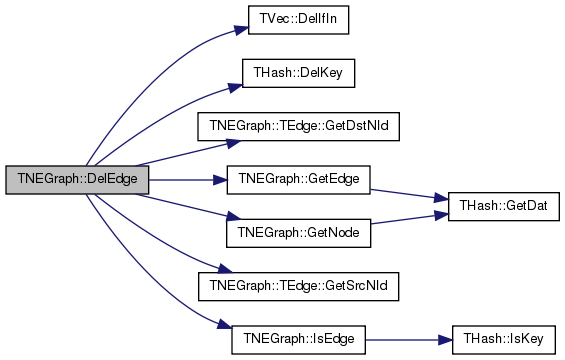void TNEGraph::DelEdge ( const int & SrcNId, const int & DstNId, const bool & IsDir = `true` )

Deletes all edges between node IDs SrcNId and DstNId from the graph.

If the edge (SrcNId, DstNId) does not exist in the graph function still completes. But the function aborts if SrcNId or DstNId are not nodes in the graph.

Definition at line 537 of file graph.cpp.

537  {
538  int EId;
539  IAssert(IsEdge(SrcNId, DstNId, EId, IsDir)); // there is at least one edge
540  while (IsEdge(SrcNId, DstNId, EId, IsDir)) {
541  GetNode(SrcNId).OutEIdV.DelIfIn(EId);
542  GetNode(DstNId).InEIdV.DelIfIn(EId);
543  }
544  EdgeH.DelKey(EId);
545 }
#define IAssert(Cond)
Definition: bd.h:262
bool DelIfIn(const TVal &Val)
Removes the first occurrence of element Val.
Definition: ds.h:1212
THash< TInt, TEdge > EdgeH
Definition: graph.h:798
void DelKey(const TKey &Key)
Definition: hash.h:404
TIntV OutEIdV
Definition: graph.h:673
TNode & GetNode(const int &NId)
Definition: graph.h:790
bool IsEdge(const int &EId) const
Tests whether an edge with edge ID EId exists in the graph.
Definition: graph.h:868
TIntV InEIdV
Definition: graph.h:673

Here is the call graph for this function: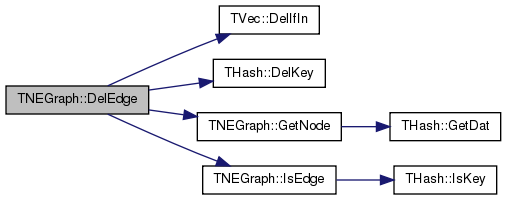void TNEGraph::DelNode ( const int & NId )

Deletes node of ID NId from the graph.

If the node of ID NId does not exist the function aborts.

Definition at line 497 of file graph.cpp.

497  {
498  const TNode& Node = GetNode(NId);
499  for (int out = 0; out < Node.GetOutDeg(); out++) {
500  const int EId = Node.GetOutEId(out);
501  const TEdge& Edge = GetEdge(EId);
502  IAssert(Edge.GetSrcNId() == NId);
503  GetNode(Edge.GetDstNId()).InEIdV.DelIfIn(EId);
504  EdgeH.DelKey(EId);
505  }
506  for (int in = 0; in < Node.GetInDeg(); in++) {
507  const int EId = Node.GetInEId(in);
508  const TEdge& Edge = GetEdge(EId);
509  IAssert(Edge.GetDstNId() == NId);
510  GetNode(Edge.GetSrcNId()).OutEIdV.DelIfIn(EId);
511  EdgeH.DelKey(EId);
512  }
513  NodeH.DelKey(NId);
514 }
#define IAssert(Cond)
Definition: bd.h:262
THash< TInt, TNode > NodeH
Definition: graph.h:797
THash< TInt, TEdge > EdgeH
Definition: graph.h:798
void DelKey(const TKey &Key)
Definition: hash.h:404
TEdge & GetEdge(const int &EId)
Definition: graph.h:792
TNode & GetNode(const int &NId)
Definition: graph.h:790

Here is the call graph for this function: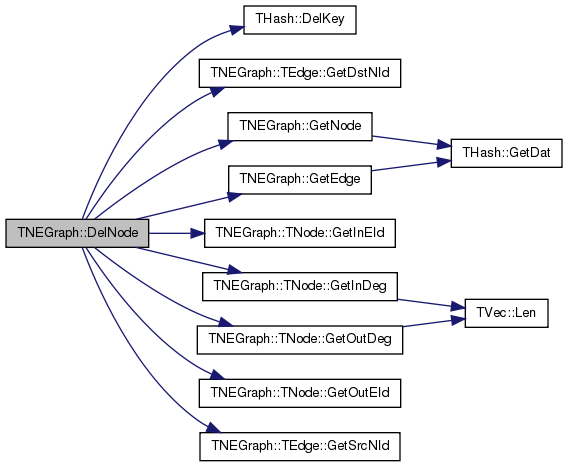void TNEGraph::DelNode ( const TNode & NodeI )
inline

Deletes node of ID NodeI.GetId() from the graph.

Definition at line 836 of file graph.h.

References DelNode(), and TNEGraph::TNode::GetId().

Referenced by DelNode().

836 { DelNode(NodeI.GetId()); }
void DelNode(const int &NId)
Deletes node of ID NId from the graph.
Definition: graph.cpp:497

Here is the call graph for this function:Here is the caller graph for this function: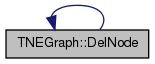void TNEGraph::Dump ( FILE * OutF = `stdout` ) const

Print the graph in a human readable form to an output stream OutF.

Definition at line 639 of file graph.cpp.

References BegEI(), BegNI(), edge, EndEI(), EndNI(), GetEdges(), and GetNodes().

639  {
640  const int NodePlaces = (int) ceil(log10((double) GetNodes()));
641  const int EdgePlaces = (int) ceil(log10((double) GetEdges()));
642  fprintf(OutF, "-------------------------------------------------\nDirected Node-Edge Graph: nodes: %d, edges: %d\n", GetNodes(), GetEdges());
643  for (TNodeI NodeI = BegNI(); NodeI < EndNI(); NodeI++) {
644  fprintf(OutF, " %*d]\n", NodePlaces, NodeI.GetId());
645  fprintf(OutF, " in[%d]", NodeI.GetInDeg());
646  for (int edge = 0; edge < NodeI.GetInDeg(); edge++) {
647  fprintf(OutF, " %*d", EdgePlaces, NodeI.GetInEId(edge)); }
648  fprintf(OutF, "\n out[%d]", NodeI.GetOutDeg());
649  for (int edge = 0; edge < NodeI.GetOutDeg(); edge++) {
650  fprintf(OutF, " %*d", EdgePlaces, NodeI.GetOutEId(edge)); }
651  fprintf(OutF, "\n");
652  }
653  for (TEdgeI EdgeI = BegEI(); EdgeI < EndEI(); EdgeI++) {
654  fprintf(OutF, " %*d] %*d -> %*d\n", EdgePlaces, EdgeI.GetId(), NodePlaces, EdgeI.GetSrcNId(), NodePlaces, EdgeI.GetDstNId());
655  }
656  fprintf(OutF, "\n");
657 }
TEdgeI BegEI() const
Returns an iterator referring to the first edge in the graph.
Definition: graph.h:876
TEdgeI EndEI() const
Returns an iterator referring to the past-the-end edge in the graph.
Definition: graph.h:878
TNodeI BegNI() const
Returns an iterator referring to the first node in the graph.
Definition: graph.h:840
TNodeI EndNI() const
Returns an iterator referring to the past-the-end node in the graph.
Definition: graph.h:842
int GetNodes() const
Returns the number of nodes in the graph.
Definition: graph.h:822
int GetEdges() const
Returns the number of edges in the graph.
Definition: graph.h:849

Here is the call graph for this function: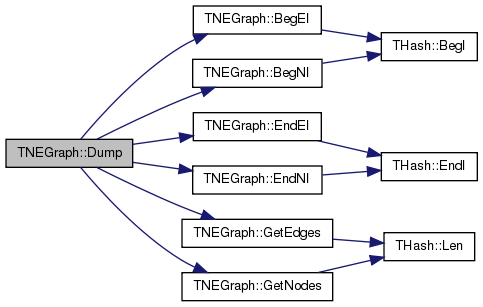bool TNEGraph::Empty ( ) const
inline

Tests whether the graph is empty (has zero nodes).

Definition at line 898 of file graph.h.

References GetNodes().

898 { return GetNodes()==0; }
int GetNodes() const
Returns the number of nodes in the graph.
Definition: graph.h:822

Here is the call graph for this function: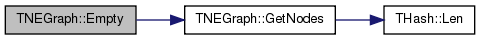TEdgeI TNEGraph::EndEI ( ) const
inline

Returns an iterator referring to the past-the-end edge in the graph.

Definition at line 878 of file graph.h.

References THash< TKey, TDat, THashFunc >::EndI().

Referenced by Dump().

878 { return TEdgeI(EdgeH.EndI(), this); }
TIter EndI() const
Definition: hash.h:218
THash< TInt, TEdge > EdgeH
Definition: graph.h:798

Here is the call graph for this function: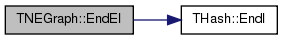Here is the caller graph for this function: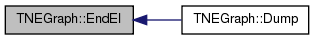TNodeI TNEGraph::EndNI ( ) const
inline

Returns an iterator referring to the past-the-end node in the graph.

Definition at line 842 of file graph.h.

References THash< TKey, TDat, THashFunc >::EndI().

Referenced by Dump(), and TBPGraph::GetEI().

842 { return TNodeI(NodeH.EndI(), this); }
TIter EndI() const
Definition: hash.h:218
THash< TInt, TNode > NodeH
Definition: graph.h:797

Here is the call graph for this function: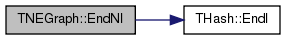Here is the caller graph for this function:TEdge& TNEGraph::GetEdge ( const int & EId )
inlineprivate

Definition at line 792 of file graph.h.

References EdgeH, and THash< TKey, TDat, THashFunc >::GetDat().

792 { return EdgeH.GetDat(EId); }
const TDat & GetDat(const TKey &Key) const
Definition: hash.h:262
THash< TInt, TEdge > EdgeH
Definition: graph.h:798

Here is the call graph for this function: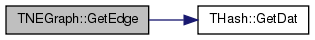Here is the caller graph for this function: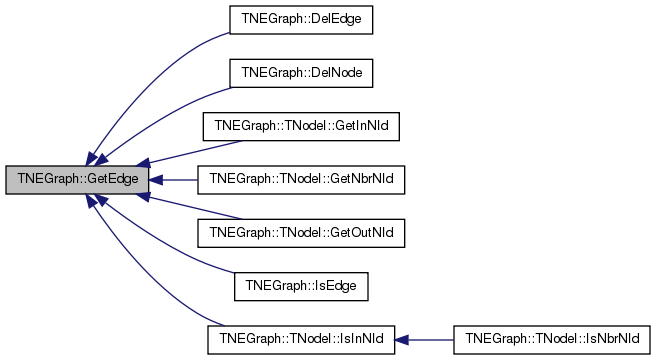const TEdge& TNEGraph::GetEdge ( const int & EId ) const
inlineprivate

Definition at line 793 of file graph.h.

References EdgeH, and THash< TKey, TDat, THashFunc >::GetDat().

793 { return EdgeH.GetDat(EId); }
const TDat & GetDat(const TKey &Key) const
Definition: hash.h:262
THash< TInt, TEdge > EdgeH
Definition: graph.h:798

Here is the call graph for this function:int TNEGraph::GetEdges ( ) const
inline

Returns the number of edges in the graph.

Definition at line 849 of file graph.h.

References THash< TKey, TDat, THashFunc >::Len().

Referenced by Dump(), TBPGraph::Dump(), and GetEIdV().

849 { return EdgeH.Len(); }
THash< TInt, TEdge > EdgeH
Definition: graph.h:798
int Len() const
Definition: hash.h:228

Here is the call graph for this function: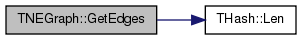Here is the caller graph for this function: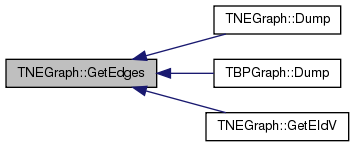TEdgeI TNEGraph::GetEI ( const int & EId ) const
inline

Returns an iterator referring to edge with edge ID EId.

Definition at line 880 of file graph.h.

References THash< TKey, TDat, THashFunc >::GetI().

Referenced by GetRndEI().

880 { return TEdgeI(EdgeH.GetI(EId), this); }
THash< TInt, TEdge > EdgeH
Definition: graph.h:798
TIter GetI(const TKey &Key) const
Definition: hash.h:220

Here is the call graph for this function: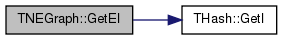Here is the caller graph for this function: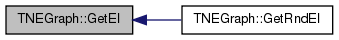TEdgeI TNEGraph::GetEI ( const int & SrcNId, const int & DstNId ) const
inline

Returns an iterator referring to edge (SrcNId, DstNId) in the graph.

Definition at line 882 of file graph.h.

References GetEI(), and GetEId().

Referenced by GetEI().

882 { return GetEI(GetEId(SrcNId, DstNId)); }
TEdgeI GetEI(const int &EId) const
Returns an iterator referring to edge with edge ID EId.
Definition: graph.h:880
int GetEId(const int &SrcNId, const int &DstNId) const
Returns an edge ID between node IDs SrcNId and DstNId, if such an edge exists. Otherwise, return -1.
Definition: graph.h:874

Here is the call graph for this function:Here is the caller graph for this function: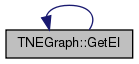int TNEGraph::GetEId ( const int & SrcNId, const int & DstNId ) const
inline

Returns an edge ID between node IDs SrcNId and DstNId, if such an edge exists. Otherwise, return -1.

Definition at line 874 of file graph.h.

References IsEdge().

Referenced by GetEI().

874 { int EId; return IsEdge(SrcNId, DstNId, EId)?EId:-1; }
bool IsEdge(const int &EId) const
Tests whether an edge with edge ID EId exists in the graph.
Definition: graph.h:868

Here is the call graph for this function: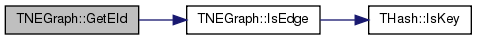Here is the caller graph for this function:void TNEGraph::GetEIdV ( TIntV & EIdV ) const

Gets a vector IDs of all edges in the graph.

Definition at line 570 of file graph.cpp.

570  {
571  EIdV.Gen(GetEdges(), 0);
572  for (int E=EdgeH.FFirstKeyId(); EdgeH.FNextKeyId(E); ) {
574  }
575 }
THash< TInt, TEdge > EdgeH
Definition: graph.h:798
bool FNextKeyId(int &KeyId) const
Definition: hash.h:478
int FFirstKeyId() const
Definition: hash.h:278
int GetEdges() const
Returns the number of edges in the graph.
Definition: graph.h:849
void Gen(const TSizeTy &_Vals)
Constructs a vector (an array) of _Vals elements.
Definition: ds.h:523
Adds a new element at the end of the vector, after its current last element.
Definition: ds.h:602
const TKey & GetKey(const int &KeyId) const
Definition: hash.h:252

Here is the call graph for this function: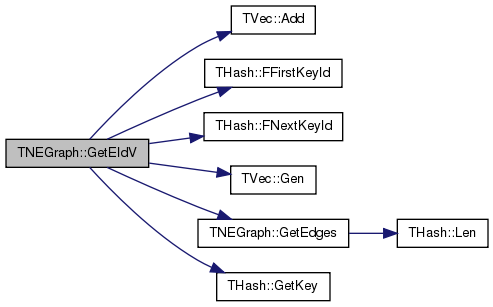int TNEGraph::GetMxNId ( ) const
inline

Returns an ID that is larger than any node ID in the graph.

Definition at line 846 of file graph.h.

References MxNId.

846 { return MxNId; }
TInt MxNId
Definition: graph.h:796
 TNodeI TNEGraph::GetNI ( const int & NId ) const
inline

Returns an iterator referring to the node of ID NId in the graph.

Definition at line 844 of file graph.h.

References THash< TKey, TDat, THashFunc >::GetI().

Referenced by TBPGraph::GetEI(), and GetRndNI().

844 { return TNodeI(NodeH.GetI(NId), this); }
THash< TInt, TNode > NodeH
Definition: graph.h:797
TIter GetI(const TKey &Key) const
Definition: hash.h:220

Here is the call graph for this function: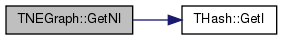Here is the caller graph for this function:void TNEGraph::GetNIdV ( TIntV & NIdV ) const

Gets a vector IDs of all nodes in the graph.

Definition at line 564 of file graph.cpp.

564  {
565  NIdV.Gen(GetNodes(), 0);
566  for (int N=NodeH.FFirstKeyId(); NodeH.FNextKeyId(N); ) {
568 }
THash< TInt, TNode > NodeH
Definition: graph.h:797
int GetNodes() const
Returns the number of nodes in the graph.
Definition: graph.h:822
bool FNextKeyId(int &KeyId) const
Definition: hash.h:478
int FFirstKeyId() const
Definition: hash.h:278
void Gen(const TSizeTy &_Vals)
Constructs a vector (an array) of _Vals elements.
Definition: ds.h:523
Adds a new element at the end of the vector, after its current last element.
Definition: ds.h:602
const TKey & GetKey(const int &KeyId) const
Definition: hash.h:252

Here is the call graph for this function: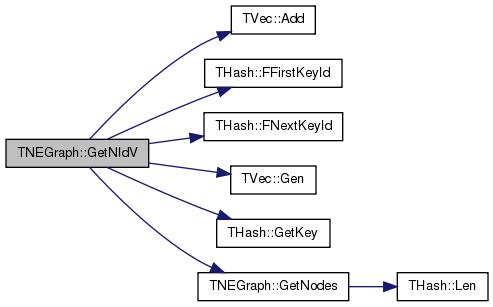TNode& TNEGraph::GetNode ( const int & NId )
inlineprivate

Definition at line 790 of file graph.h.

References THash< TKey, TDat, THashFunc >::GetDat(), and NodeH.

Referenced by AddEdge(), DelEdge(), DelNode(), and IsEdge().

790 { return NodeH.GetDat(NId); }
const TDat & GetDat(const TKey &Key) const
Definition: hash.h:262
THash< TInt, TNode > NodeH
Definition: graph.h:797

Here is the call graph for this function: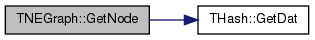Here is the caller graph for this function: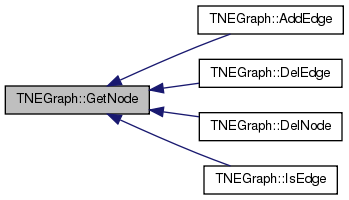const TNode& TNEGraph::GetNode ( const int & NId ) const
inlineprivate

Definition at line 791 of file graph.h.

References THash< TKey, TDat, THashFunc >::GetDat(), and NodeH.

791 { return NodeH.GetDat(NId); }
const TDat & GetDat(const TKey &Key) const
Definition: hash.h:262
THash< TInt, TNode > NodeH
Definition: graph.h:797

Here is the call graph for this function:int TNEGraph::GetNodes ( ) const
inline

Returns the number of nodes in the graph.

Definition at line 822 of file graph.h.

References THash< TKey, TDat, THashFunc >::Len().

Referenced by Dump(), TBPGraph::Dump(), Empty(), GetNIdV(), TBPGraph::GetNIdV(), and TBPGraph::GetRndNId().

822 { return NodeH.Len(); }
THash< TInt, TNode > NodeH
Definition: graph.h:797
int Len() const
Definition: hash.h:228

Here is the call graph for this function: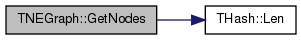Here is the caller graph for this function: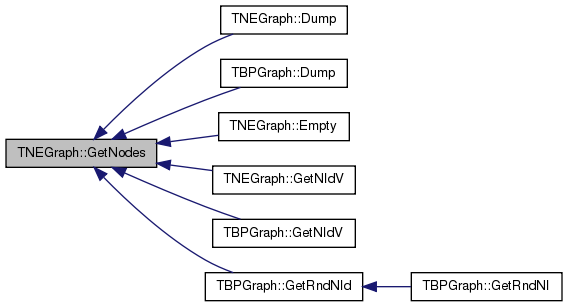TEdgeI TNEGraph::GetRndEI ( TRnd & Rnd = `TInt::Rnd` )
inline

Returns an interator referring to a random edge in the graph.

Definition at line 891 of file graph.h.

References GetEI(), and GetRndEId().

891 { return GetEI(GetRndEId(Rnd)); }
TEdgeI GetEI(const int &EId) const
Returns an iterator referring to edge with edge ID EId.
Definition: graph.h:880
int GetRndEId(TRnd &Rnd=TInt::Rnd)
Returns an ID of a random edge in the graph.
Definition: graph.h:889

Here is the call graph for this function:int TNEGraph::GetRndEId ( TRnd & Rnd = `TInt::Rnd` )
inline

Returns an ID of a random edge in the graph.

Definition at line 889 of file graph.h.

Referenced by GetRndEI().

889 { return EdgeH.GetKey(EdgeH.GetRndKeyId(Rnd, 0.8)); }
THash< TInt, TEdge > EdgeH
Definition: graph.h:798
int GetRndKeyId(TRnd &Rnd) const
Get an index of a random element. If the hash table has many deleted keys, this may take a long time...
Definition: hash.h:444
const TKey & GetKey(const int &KeyId) const
Definition: hash.h:252

Here is the call graph for this function:Here is the caller graph for this function: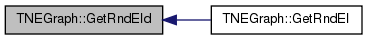TNodeI TNEGraph::GetRndNI ( TRnd & Rnd = `TInt::Rnd` )
inline

Returns an interator referring to a random node in the graph.

Definition at line 887 of file graph.h.

References GetNI(), and GetRndNId().

887 { return GetNI(GetRndNId(Rnd)); }
TNodeI GetNI(const int &NId) const
Returns an iterator referring to the node of ID NId in the graph.
Definition: graph.h:844
int GetRndNId(TRnd &Rnd=TInt::Rnd)
Returns an ID of a random node in the graph.
Definition: graph.h:885

Here is the call graph for this function:int TNEGraph::GetRndNId ( TRnd & Rnd = `TInt::Rnd` )
inline

Returns an ID of a random node in the graph.

Definition at line 885 of file graph.h.

Referenced by GetRndNI().

885 { return NodeH.GetKey(NodeH.GetRndKeyId(Rnd, 0.8)); }
THash< TInt, TNode > NodeH
Definition: graph.h:797
int GetRndKeyId(TRnd &Rnd) const
Get an index of a random element. If the hash table has many deleted keys, this may take a long time...
Definition: hash.h:444
const TKey & GetKey(const int &KeyId) const
Definition: hash.h:252

Here is the call graph for this function:Here is the caller graph for this function: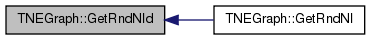PNEGraph TNEGraph::GetSmallGraph ( )
static

Returns a small multigraph on 5 nodes and 6 edges.

```/// Edges:  0 -> 1, 0 -> 2, 0 -> 3, 0 -> 4, 1 -> 2, 1 -> 2
/// ```

Definition at line 660 of file graph.cpp.

References New().

660  {
661  PNEGraph Graph = TNEGraph::New();
662  for (int i = 0; i < 5; i++) { Graph->AddNode(i); }
666  return Graph;
667 }
static PNEGraph New()
Static constructor that returns a pointer to the graph. Call: PNEGraph Graph = TNEGraph::New().
Definition: graph.h:809
Definition: bd.h:196

Here is the call graph for this function: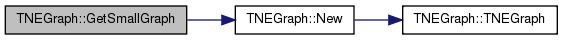bool TNEGraph::HasFlag ( const TGraphFlag & Flag ) const

Allows for run-time checking the type of the graph (see the TGraphFlag for flags).

Definition at line 464 of file graph.cpp.

References HasGraphFlag.

464  {
465  return HasGraphFlag(TNEGraph::TNet, Flag);
466 }
Directed multigraph.
Definition: graph.h:665
#define HasGraphFlag(TGraph, Flag)
For quick testing of the properties of the graph/network object (see TGraphFlag). ...
Definition: gbase.h:41
 bool TNEGraph::IsEdge ( const int & EId ) const
inline

Tests whether an edge with edge ID EId exists in the graph.

Definition at line 868 of file graph.h.

References THash< TKey, TDat, THashFunc >::IsKey().

Referenced by AddEdge(), DelEdge(), GetEId(), and IsOk().

868 { return EdgeH.IsKey(EId); }
THash< TInt, TEdge > EdgeH
Definition: graph.h:798
bool IsKey(const TKey &Key) const
Definition: hash.h:258

Here is the call graph for this function: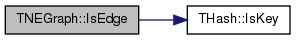Here is the caller graph for this function: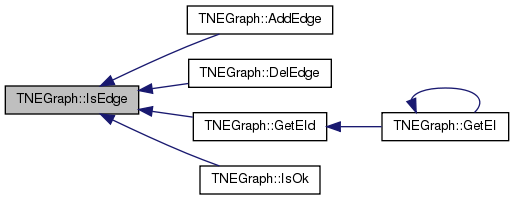bool TNEGraph::IsEdge ( const int & SrcNId, const int & DstNId, const bool & IsDir = `true` ) const
inline

Tests whether an edge between node IDs SrcNId and DstNId exists in the graph.

Definition at line 870 of file graph.h.

References IsEdge().

Referenced by IsEdge().

870 { int EId; return IsEdge(SrcNId, DstNId, EId, IsDir); }
bool IsEdge(const int &EId) const
Tests whether an edge with edge ID EId exists in the graph.
Definition: graph.h:868

Here is the call graph for this function: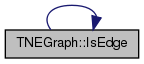Here is the caller graph for this function: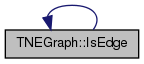bool TNEGraph::IsEdge ( const int & SrcNId, const int & DstNId, int & EId, const bool & IsDir = `true` ) const

Tests whether an edge between node IDs SrcNId and DstNId exists in the graph. if an edge exists, return its edge ID in EId.

Definition at line 547 of file graph.cpp.

547  {
548  const TNode& SrcNode = GetNode(SrcNId);
549  for (int edge = 0; edge < SrcNode.GetOutDeg(); edge++) {
550  const TEdge& Edge = GetEdge(SrcNode.GetOutEId(edge));
551  if (DstNId == Edge.GetDstNId()) {
552  EId = Edge.GetId(); return true; }
553  }
554  if (! IsDir) {
555  for (int edge = 0; edge < SrcNode.GetInDeg(); edge++) {
556  const TEdge& Edge = GetEdge(SrcNode.GetInEId(edge));
557  if (DstNId == Edge.GetSrcNId()) {
558  EId = Edge.GetId(); return true; }
559  }
560  }
561  return false;
562 }
TEdge & GetEdge(const int &EId)
Definition: graph.h:792
TNode & GetNode(const int &NId)
Definition: graph.h:790
int GetId() const
Definition: graph.h:700

Here is the call graph for this function: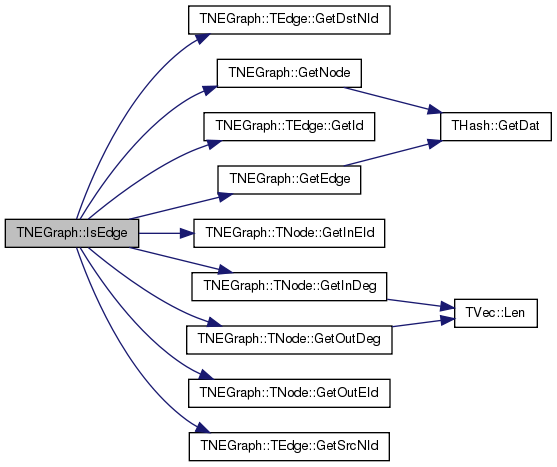bool TNEGraph::IsNode ( const int & NId ) const
inline

Tests whether ID NId is a node.

Definition at line 838 of file graph.h.

References THash< TKey, TDat, THashFunc >::IsKey().

838 { return NodeH.IsKey(NId); }
THash< TInt, TNode > NodeH
Definition: graph.h:797
bool IsKey(const TKey &Key) const
Definition: hash.h:258

Here is the call graph for this function: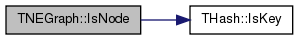Here is the caller graph for this function: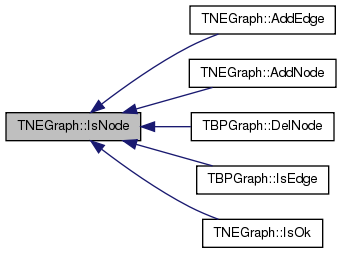bool TNEGraph::IsOk ( const bool & ThrowExcept = `true` ) const

Checks the graph data structure for internal consistency.

For each node in the graph check that its neighbors are also nodes in the graph.

Definition at line 586 of file graph.cpp.

586  {
587 bool RetVal = true;
588  for (int N = NodeH.FFirstKeyId(); NodeH.FNextKeyId(N); ) {
589  const TNode& Node = NodeH[N];
590  if (! Node.OutEIdV.IsSorted()) {
591  const TStr Msg = TStr::Fmt("Out-edge list of node %d is not sorted.", Node.GetId());
592  if (ThrowExcept) { EAssertR(false, Msg); } else { ErrNotify(Msg.CStr()); } RetVal=false;
593  }
594  if (! Node.InEIdV.IsSorted()) {
595  const TStr Msg = TStr::Fmt("In-edge list of node %d is not sorted.", Node.GetId());
596  if (ThrowExcept) { EAssertR(false, Msg); } else { ErrNotify(Msg.CStr()); } RetVal=false;
597  }
598  // check out-edge ids
599  int prevEId = -1;
600  for (int e = 0; e < Node.GetOutDeg(); e++) {
601  if (! IsEdge(Node.GetOutEId(e))) {
602  const TStr Msg = TStr::Fmt("Out-edge id %d of node %d does not exist.", Node.GetOutEId(e), Node.GetId());
603  if (ThrowExcept) { EAssertR(false, Msg); } else { ErrNotify(Msg.CStr()); } RetVal=false;
604  }
605  if (e > 0 && prevEId == Node.GetOutEId(e)) {
606  const TStr Msg = TStr::Fmt("Node %d has duplidate out-edge id %d.", Node.GetId(), Node.GetOutEId(e));
607  if (ThrowExcept) { EAssertR(false, Msg); } else { ErrNotify(Msg.CStr()); } RetVal=false;
608  }
609  prevEId = Node.GetOutEId(e);
610  }
611  // check in-edge ids
612  prevEId = -1;
613  for (int e = 0; e < Node.GetInDeg(); e++) {
614  if (! IsEdge(Node.GetInEId(e))) {
615  const TStr Msg = TStr::Fmt("Out-edge id %d of node %d does not exist.", Node.GetInEId(e), Node.GetId());
616  if (ThrowExcept) { EAssertR(false, Msg); } else { ErrNotify(Msg.CStr()); } RetVal=false;
617  }
618  if (e > 0 && prevEId == Node.GetInEId(e)) {
619  const TStr Msg = TStr::Fmt("Node %d has duplidate out-edge id %d.", Node.GetId(), Node.GetInEId(e));
620  if (ThrowExcept) { EAssertR(false, Msg); } else { ErrNotify(Msg.CStr()); } RetVal=false;
621  }
622  prevEId = Node.GetInEId(e);
623  }
624  }
625  for (int E = EdgeH.FFirstKeyId(); EdgeH.FNextKeyId(E); ) {
626  const TEdge& Edge = EdgeH[E];
627  if (! IsNode(Edge.GetSrcNId())) {
628  const TStr Msg = TStr::Fmt("Edge %d source node %d does not exist.", Edge.GetId(), Edge.GetSrcNId());
629  if (ThrowExcept) { EAssertR(false, Msg); } else { ErrNotify(Msg.CStr()); } RetVal=false;
630  }
631  if (! IsNode(Edge.GetDstNId())) {
632  const TStr Msg = TStr::Fmt("Edge %d destination node %d does not exist.", Edge.GetId(), Edge.GetDstNId());
633  if (ThrowExcept) { EAssertR(false, Msg); } else { ErrNotify(Msg.CStr()); } RetVal=false;
634  }
635  }
636  return RetVal;
637 }
void ErrNotify(const char *NotifyCStr)
Definition: bd.h:74
THash< TInt, TNode > NodeH
Definition: graph.h:797
THash< TInt, TEdge > EdgeH
Definition: graph.h:798
bool FNextKeyId(int &KeyId) const
Definition: hash.h:478
int FFirstKeyId() const
Definition: hash.h:278
bool IsEdge(const int &EId) const
Tests whether an edge with edge ID EId exists in the graph.
Definition: graph.h:868
Definition: dt.h:412
static TStr Fmt(const char *FmtStr,...)
Definition: dt.cpp:1599
#define EAssertR(Cond, MsgStr)
Definition: bd.h:283
char * CStr()
Definition: dt.h:479
bool IsNode(const int &NId) const
Tests whether ID NId is a node.
Definition: graph.h:838

Here is the call graph for this function: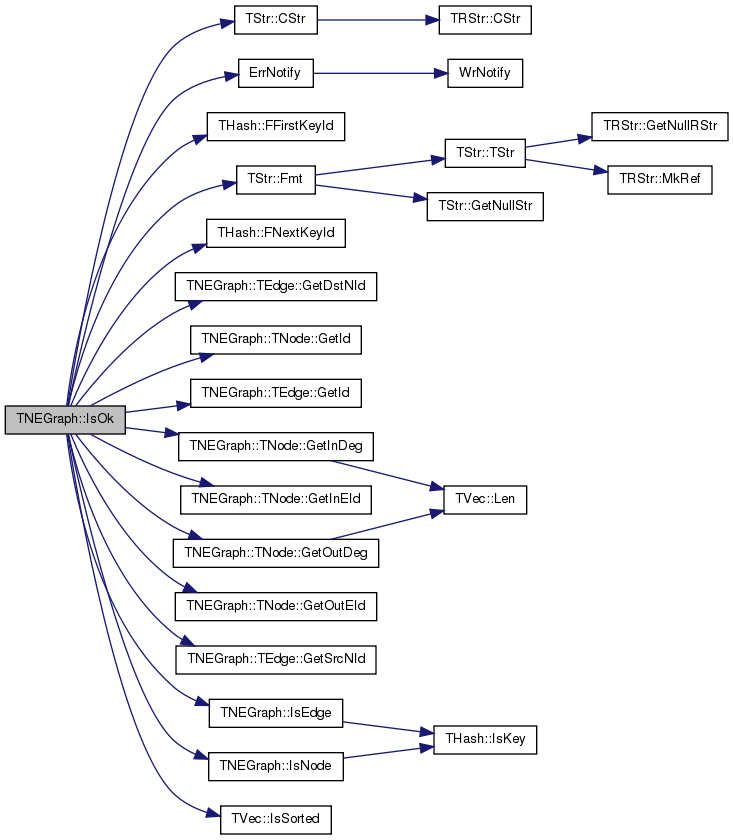static PNEGraph TNEGraph::Load ( TSIn & SIn )
inlinestatic

Static constructor that loads the graph from a stream SIn and returns a pointer to it.

Definition at line 815 of file graph.h.

References TNEGraph().

815 { return PNEGraph(new TNEGraph(SIn)); }
TPt< TNEGraph > PNEGraph
Pointer to a directed multigraph (TNEGraph)
Definition: graph.h:21
TNEGraph()
Definition: graph.h:800

Here is the call graph for this function: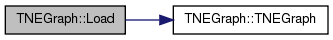static PNEGraph TNEGraph::New ( )
inlinestatic

Static constructor that returns a pointer to the graph. Call: PNEGraph Graph = TNEGraph::New().

Definition at line 809 of file graph.h.

References TNEGraph().

Referenced by GetSmallGraph().

809 { return PNEGraph(new TNEGraph()); }
TPt< TNEGraph > PNEGraph
Pointer to a directed multigraph (TNEGraph)
Definition: graph.h:21
TNEGraph()
Definition: graph.h:800

Here is the call graph for this function:Here is the caller graph for this function: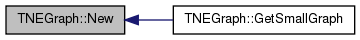static PNEGraph TNEGraph::New ( const int & Nodes, const int & Edges )
inlinestatic

Static constructor that returns a pointer to the graph and reserves enough memory for Nodes nodes and Edges edges.

Call: PNEGraph Graph = TNEGraph::New(Nodes, Edges).

Definition at line 813 of file graph.h.

References TNEGraph().

813 { return PNEGraph(new TNEGraph(Nodes, Edges)); }
TPt< TNEGraph > PNEGraph
Pointer to a directed multigraph (TNEGraph)
Definition: graph.h:21
TNEGraph()
Definition: graph.h:800

Here is the call graph for this function: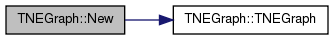TNEGraph& TNEGraph::operator= ( const TNEGraph & Graph )
inline

Definition at line 818 of file graph.h.

References EdgeH, MxEId, MxNId, and NodeH.

818  { if (this!=&Graph) {
819  MxNId=Graph.MxNId; MxEId=Graph.MxEId; NodeH=Graph.NodeH; EdgeH=Graph.EdgeH; } return *this; }
THash< TInt, TNode > NodeH
Definition: graph.h:797
THash< TInt, TEdge > EdgeH
Definition: graph.h:798
TInt MxNId
Definition: graph.h:796
TInt MxEId
Definition: graph.h:796
 void TNEGraph::Reserve ( const int & Nodes, const int & Edges )
inline

Reserves memory for a graph of Nodes nodes and Edges edges.

Definition at line 902 of file graph.h.

References THash< TKey, TDat, THashFunc >::Gen().

Referenced by TNEGraph().

902  {
903  if (Nodes>0) { NodeH.Gen(Nodes/2); } if (Edges>0) { EdgeH.Gen(Edges/2); } }
THash< TInt, TNode > NodeH
Definition: graph.h:797
THash< TInt, TEdge > EdgeH
Definition: graph.h:798
void Gen(const int &ExpectVals)
Definition: hash.h:222

Here is the call graph for this function: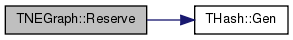Here is the caller graph for this function: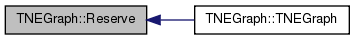void TNEGraph::Save ( TSOut & SOut ) const
inline

Saves the graph to a (binary) stream SOut.

Definition at line 807 of file graph.h.

References THash< TKey, TDat, THashFunc >::Save(), and TInt::Save().

807 { MxNId.Save(SOut); MxEId.Save(SOut); NodeH.Save(SOut); EdgeH.Save(SOut); }
void Save(TSOut &SOut) const
Definition: dt.h:1153
void Save(TSOut &SOut) const
Definition: hash.h:183
THash< TInt, TNode > NodeH
Definition: graph.h:797
THash< TInt, TEdge > EdgeH
Definition: graph.h:798
TInt MxNId
Definition: graph.h:796
TInt MxEId
Definition: graph.h:796

Here is the call graph for this function:## Friends And Related Function Documentation

 friend class TPt< TNEGraph >
friend

Definition at line 924 of file graph.h.

## Member Data Documentation

 TCRef TNEGraph::CRef
private

Definition at line 795 of file graph.h.

 THash TNEGraph::EdgeH
private

Definition at line 798 of file graph.h.

Referenced by AddEdge(), Defrag(), DelEdge(), DelNode(), GetEdge(), GetEIdV(), IsOk(), and operator=().

 TInt TNEGraph::MxEId
private

Definition at line 796 of file graph.h.

 TInt TNEGraph::MxNId
private

Definition at line 796 of file graph.h.